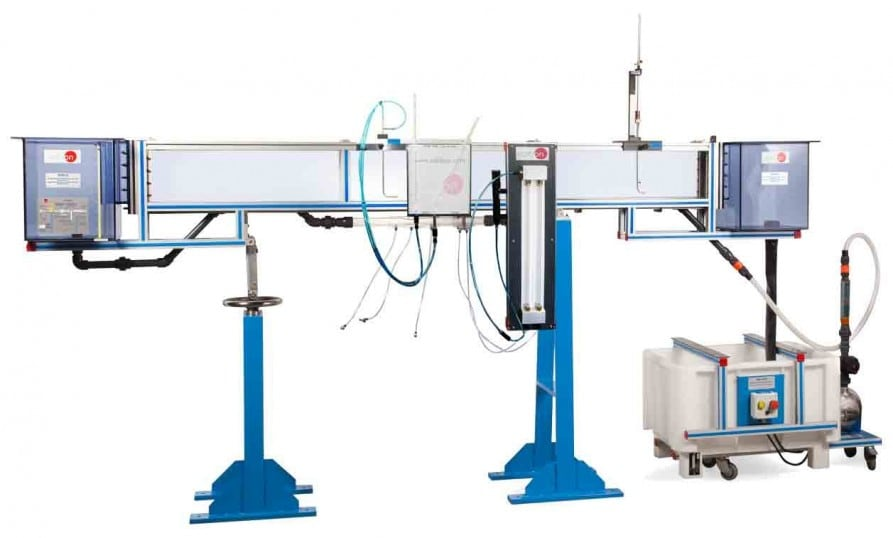# CF 流路（流路面積：80mm×300mm）## 概要

Channel, through which water flows, of rectangular section with transparent walls that enable to observe all the experiments. Water is taken from the storage tank by means of a hydraulic pump and, by the pipe, it is driven to the inlet tank, where there is a flow stilling device. After that, the water flows through the channel that discharges in the reception tank. Finally it comes back to the storage tank, completing the closed circuit.

To regulate the flow through the channel, there is a valve at the output of the pump.

To measure the flow there is an orifice plate flowmeter and a Venturi type flowmeter. The flow also can be measured with the flowmeter of the Basic Hydraulic Feed System (FME00/B).

A limnimeter (CFRM) is required to measure water level. Besides, a Pitot tube with tubes manometer board (CFTP) us required to measure velocity/flow.

The channel is assembled on two supports, with a system to control the inclination of the channel.

There is a wide range of available accessories.

## 演習と指導の慣行

### マニュアルに含まれるガイド付き実習

1. Measurement of water level and speed along the channel.
2. Flow rate measurement using sharp-crested weirs.
3. Flow rate measurement through changes in the channel section.
4. Flow rate measurement using Venturi flume.
5. Flow rate control using gates.
6. Level control using syphons.
7. Flow over overflow weirs.
8. Flow between the piers of a bridge.
9. Connection of a channel to a culvert.
10. Characterization of the hydraulic jump.
11. Profiles of the water free surface.
12. Manning coefficient calculation for channels with sedimentation.
13. Comparison of the discharge coefficient in gates with or without sedimentation.
14. Simulation of the effect of the piers of a bridge in a channel with sediments.
15. Study of turbulences using ink.
16. Visualization of the transitions of the sediments on the bed when abrupt discharges are generated.
17. Dependence of the sediments shape on the flow rate variation.
18. Study of the sediments transport and settling mechanisms.

### より実用的な練習をして、ユニットを完成させる

1. Investigation of currents and torrential floods states.
2. Water levels measurement.
3. Discharge process in a submergible spillway.
4. Pressure drop in open channels.
5. Operation and study of a syphon.
6. Flow and discharge coefficient of a syphon.
7. Flow in pipes.
8. Comparison between overflow and syphon.
9. Study of the amplitude of the hydraulic jump.
10. Generation of different flow states using an underwater dam.
11. Study of the discharge processes under an adjustable weir:
• Study of alternating changes during the discharge.
1. Relationship between backwater level and discharge level.
2. Study of discharge under a radial gate:
• Study of alternating changes during the discharge.
1. Hydrostatic pressure on a weir.
2. Study of waves.
3. Behaviour of structures under swell conditions (in rough sea).
4. Application and understanding of Manning formula.
5. Study of subcritical and supercritical flows.
6. Learning how to apply the force, momentum and energy equations in typical situations.
7. Study of the transition from flowing current to accelerated current.
8. Calculation of the water flow.
9. Use of the limnimeter. To determine the operating characteristics of a Francis turbine at different speeds.

#### 品質#### アフターサービスリクエスト情報